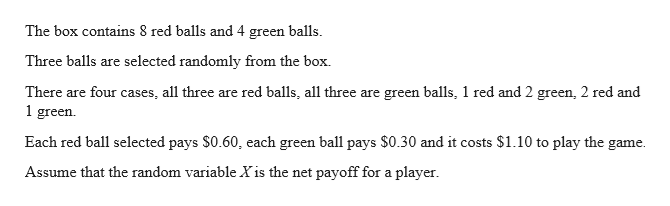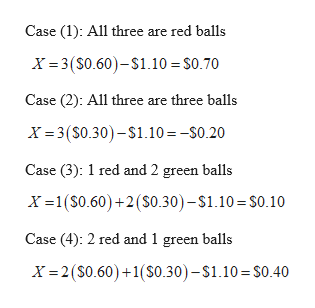# In which three balls are selected simultaneously and randomly from a box. But assume that the box contains 8 red balls and 4 green balls. Also assume that each red ball selected pays \$0.60, that each green ball selected pays \$0.30, and that it costs \$1.10 to play the game. A random variable XX is defined to be the net payoff for a player. How many different values are possible for the random variable XX?

Question
2 views

In which three balls are selected simultaneously and randomly from a box. But assume that the box contains 8 red balls and 4 green balls. Also assume that each red ball selected pays \$0.60, that each green ball selected pays \$0.30, and that it costs \$1.10 to play the game. A random variable XX is defined to be the net payoff for a player. How many different values are possible for the random variable XX?

check_circle

Step 1help_outlineImage TranscriptioncloseThe box contains 8 red balls and 4 green balls. Three balls are selected randomly from the box. There are four cases, all three are red balls, all three are green balls, 1 red and 2 green, 2 red and 1 green. Each red ball selected pays \$0.60, each green ball pays \$0.30 and it costs \$1.10 to play the game. Assume that the random variable X is the net payoff for a player. fullscreen
Step 2help_outlineImage TranscriptioncloseCase (1): All three are red balls X = 3(S0.60)-\$1.10 = \$0.70 Case (2): All three are three balls X = 3(S0.30)– S1.10 = -S0.20 Case (3): 1 red and 2 green balls X =1(S0.60)+2(S0.30)-\$1.10 = S0.10 Case (4): 2 red and 1 green balls X = 2(S0.60)+1(S0.30)-\$1.10 = S0.40 fullscreen

### Want to see the full answer?

See Solution

#### Want to see this answer and more?

Solutions are written by subject experts who are available 24/7. Questions are typically answered within 1 hour.*

See Solution
*Response times may vary by subject and question.
Tagged in

### Math